# Intermediate Maths Solutions for Locus

Inter Mathematics 1b solutions for textbook Chapter 1 Locus exercise 1(a) are given.

These solutions are very easy to understand. Study the textbook lesson very well.

Observe the example problems and solutions given in the textbook.

Observe the given solutions and try them in your own method

You can see the solutions for text book Maths 1A

Functions

Exercise 1(a)

Exercise 1(b)

Exercise 1(c)

Mathematical Induction

Exercise 2(a)

Matrices

Exercise 3(a)

Exercise 3(b)

Exercise 3(c)

Exercise 3(d)

Exercise 3(e)

Exercise 3(f)

Exercise 3(g)

Exercise 3(h)

Exercise 3(i)

Exercise 4(a)

Exercise 4(b)

Product of vectors

Exercise 5(a)

Exercise 5(b)

Exercise 5(c)

Trigonometric Ratios up to Transformations

Exercise 6(a)

Exercise 6(b)

Exercise 6(c)

Exercise 6(d)

Exercise 6(e)

Exercise 6(f)

Trigonometric Equations

Exercise 7(a)

Inverse Trigonometric Equations

Exercise 8(a)

Hyperbolic Functions

Exercise 9(a)

Properties of Triangles

Exercise 10(a)

Exercisr 10(b)

Maths 1B solutions for

Locus

Exercise 1(a)

Transformation of Axes

Exercise 2(a)

Three Dimensional Coordinates

Exercise 5(a)
Exercise 5(b)
The Plane

Exercise 7(a)
Limits and Continuity

Exercise 8(a)

Exercise 8(b)

M

# Maths 1b Inter Solutions for Locus Exercise 1(a)

Chapter 1 solutions

Locus Exercise 1(a)

Locus Exercise 1(a) Problem I Question 1Locus Exercise 1(a) Problem I Question 2Locus Exercise 1(a) Problem I Question 3Locus Exercise 1(a) Problem I Question 4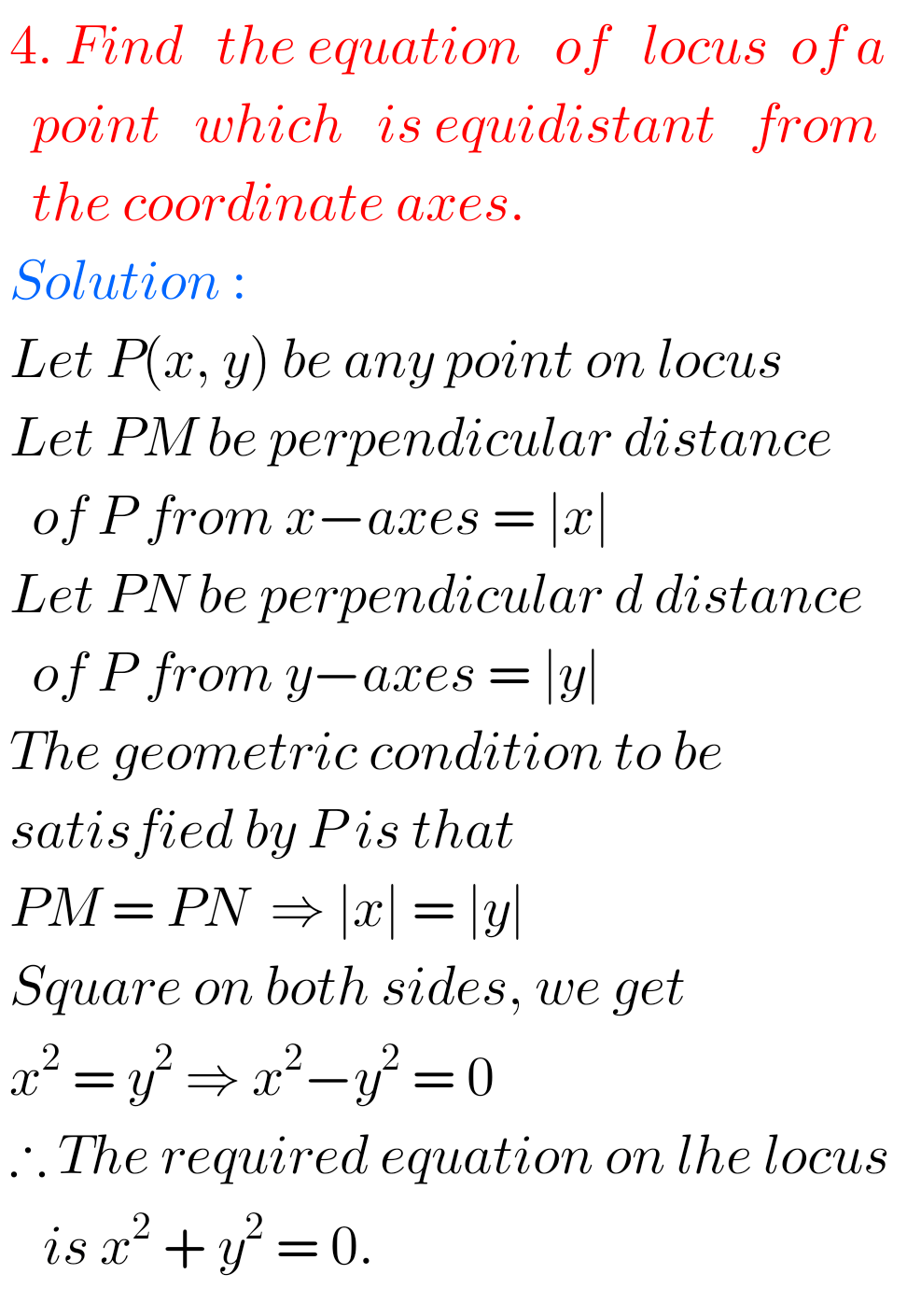Locus Exercise 1(a) Problem I Question 5Locus Exercise 1(a) Problem I Question 6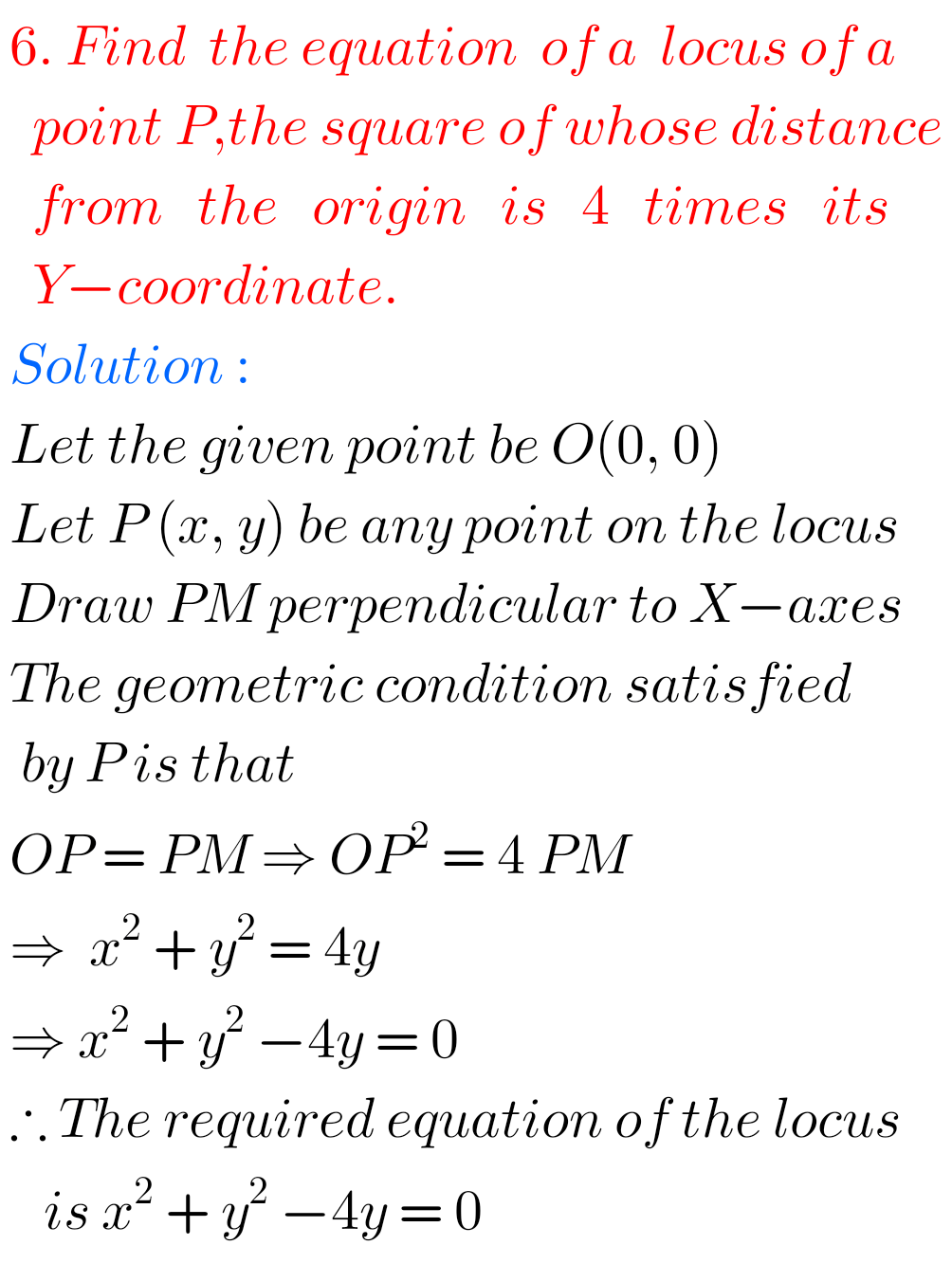Locus Exercise 1(a) Problem I Question 7## Locus solutions Inter Maths 1b exercise 1(a)

Locus Exercise 1(a) Problem II Question 1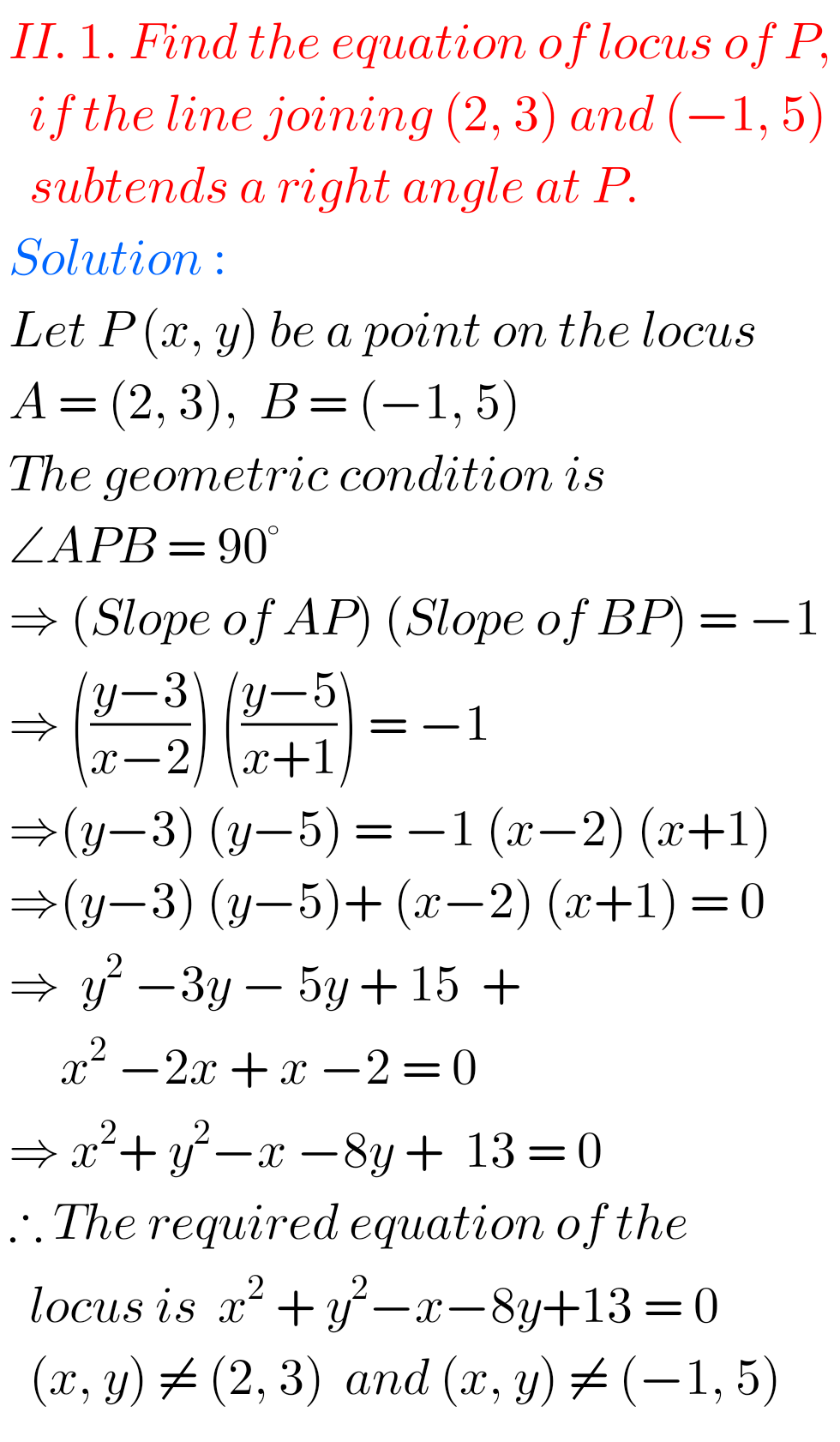Locus Exercise 1(a) Problem II Question 2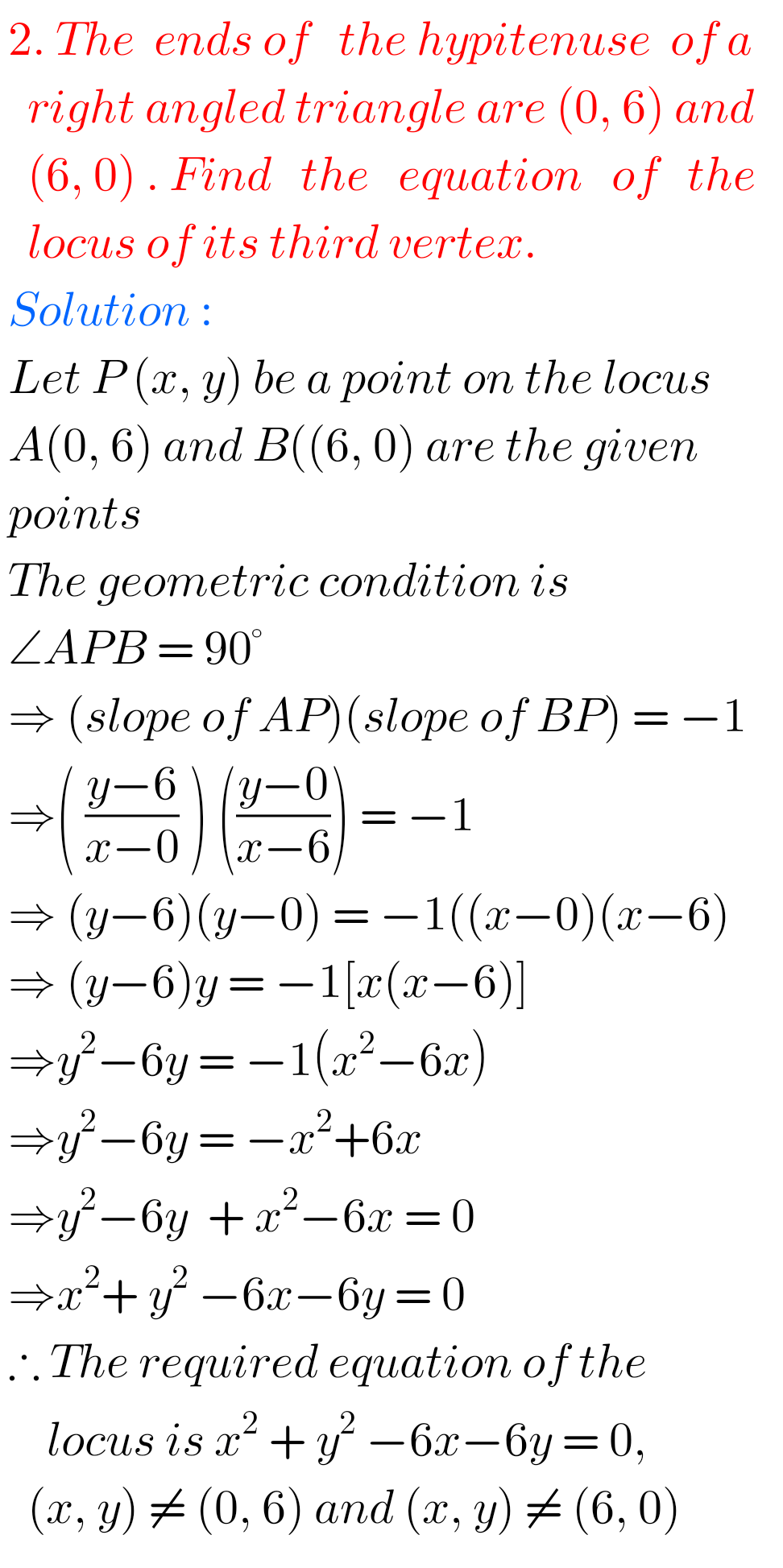Locus Exercise 1(a) Problem II Question 3Locus Exercise 1(a) Problem II Question 4Locus Exercise 1(a) Problem II Question 5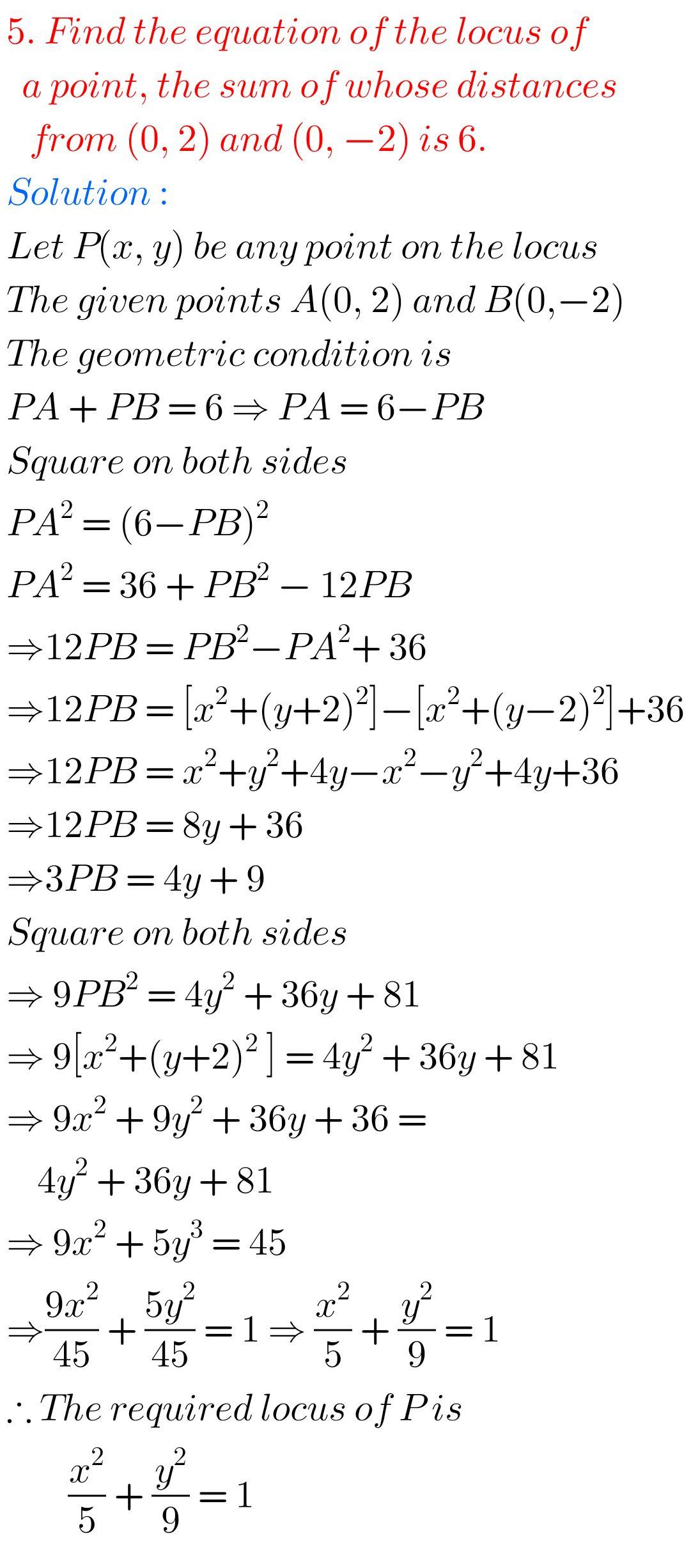## Solutions for Locus Maths Inter exercise 1(a)

Locus Exercise 1(a) Problem II Question 6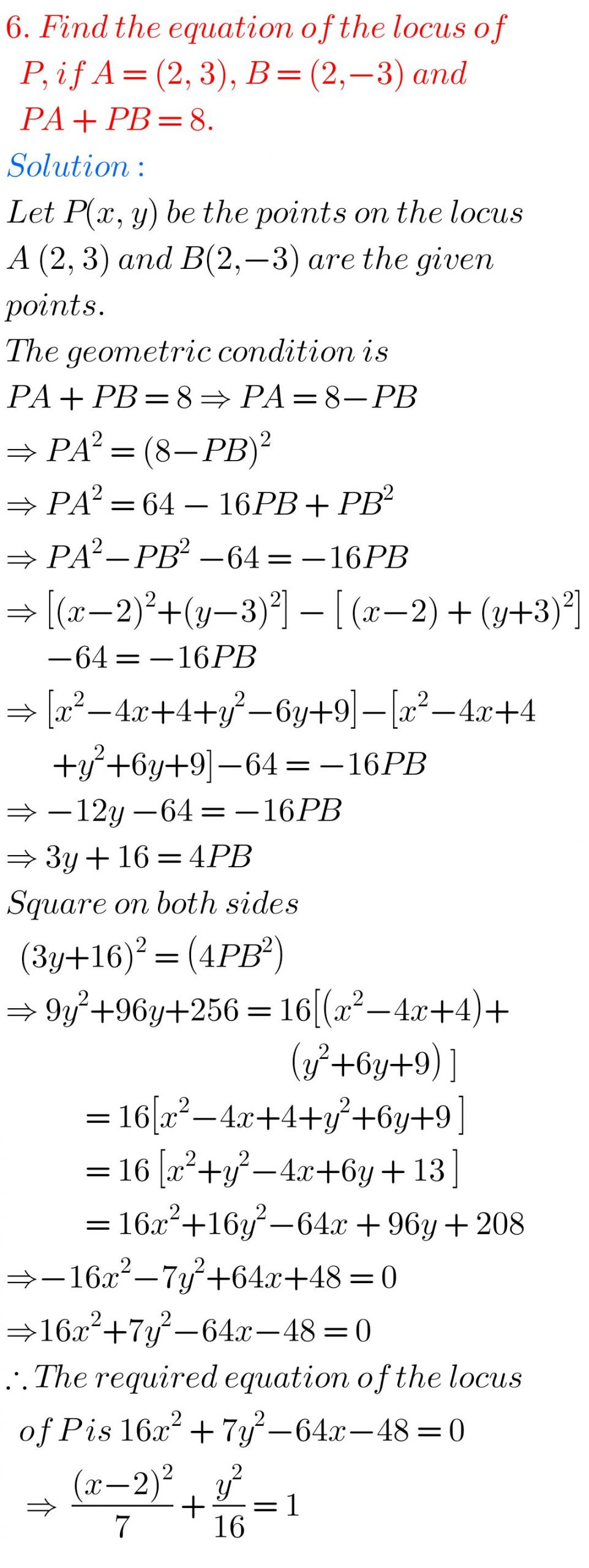Locus Exercise 1(a) Problem I Question 7Locus Exercise 1(a) Problem II Question 8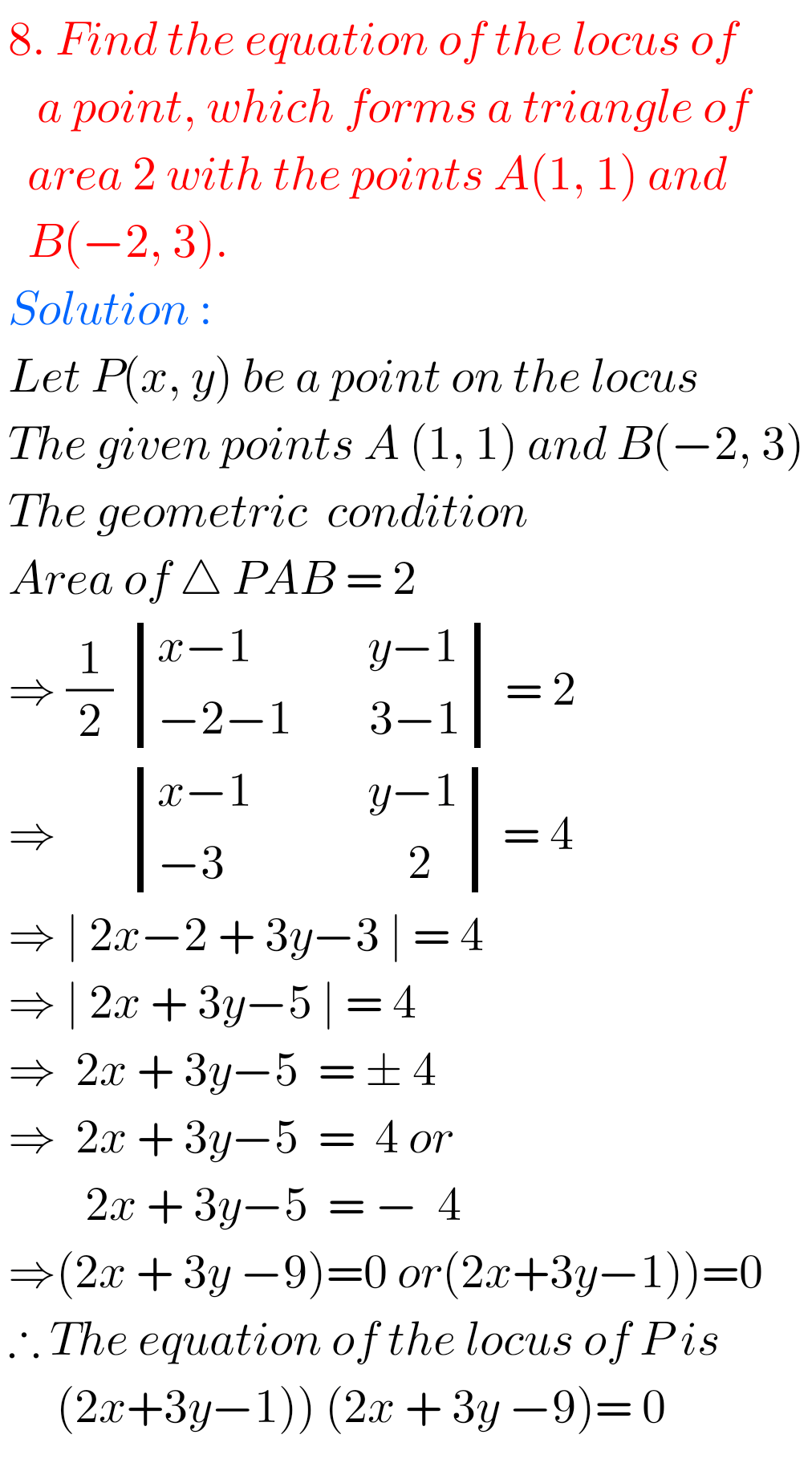Locus Exercise 1(a) Problem II Question 9Locus Exercise 1(a) Problem II Question 10M

Note ; Observe the solutions and try them in your own method.

You can also see

SSC Maths text book Solutions class 10

Inter Maths 1A solutions

Inter Maths 1B solutions

Inter Maths 1IA text book solutions

Inter Maths IIB text book solutions

You can see the solutions for junior inter maths 1A for examination purpose

2. Matrices

Junior inter 1B solutions of Examination purpose

1Locus

Straight lines sa

Straight lines la

12. Rate measure